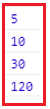# Promise Chaining in JavaScript

## JavaScript Promise Chaining with Examples

In this article, I am going to discuss the JavaScript Promise Chaining with Examples. Please read our previous article where we discussed the basics of JavaScript Promises in detail.

##### Promise Chaining in JavaScript

The methods of the Promise object such as promise.then(), promise.catch() and promise.finally() are used to connect further actions with a promise that becomes settled. These methods also return a separate newly generated promise object. Therefore, we can call the promise’s instance method on the returned Promise. Thus, calling methods in this way is referred to as the promise chaining

The promise chain is used to executes multiple asynchronous tasks in sequence. Let’s see the example first where we First, create a new promise that resolves to the value 5 after 3 seconds:

```<html>
<title>JavaScript Promise chaining example</title>
<body>
<script>
let p = new Promise((resolve, reject) => {
setTimeout(() => {
resolve(5);
}, 3 * 1000); // (*)
});

p.then((result) => { //(**)
console.log(result); // 5
return result * 2;
}).then((result) => { //(***)
console.log(result); // 10
return result * 3;
}).then((result) => {
console.log(result); // 30
return result * 4;
}).then((result) => {
console.log(result); // 120
return result * 5;
});
</script>
</body>
</html>
```

Output: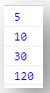Note that we have used the setTimeout() method to simulate an asynchronous operation. Then we invoke the then() method on the promise. The logic is that the result is passed through the chain of .then() handlers.

Because the then() method of a promise returns a new Promise whose promise resolves to the return value, we can call the then() method on the returned Promise. Returning a Promise from a then() callback will append it to the promise chain.

The then() method returns a Promise, even if one or both of the handler functions are absent. Because of this, multiple .then() methods can be chained together. This is known as composition.

##### Here the flow of the above example is:
1. The initial promise resolves 5 in 3 seconds (with comment line *),
2. Then the .then function is called (**).
3. The value that it returns is passed to the next then function (***)
4. …and so on.

Since the result is passed along the chain of.then() handlers, we can see a sequence of console.log calls: 5 → 10 → 30 → 120

##### The following picture illustrates the promise chaining: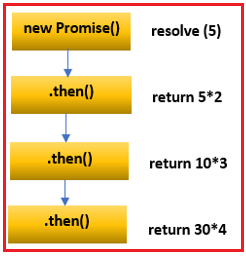Like then() method, a catch() method allows a rejected promise to recover, similar to how to catch in a try/catch statement works. Any chained then() after a catch() will execute its resolve handler using the value resolved from the catch. See the following example that elaborate it more clearly

##### Example: JavaScript Promise chaining then() after catch() method
```<html>
<title>JavaScript Promise chaining then() after catch() method example</title>
<body>
<script>
const p = new Promise(
resolve => {
throw 'oh no error occured'
});

p.catch(() => 'oh yes')
.then(console.log.bind(console)); // output "oh yes"
</script>
</body>
</html>
```

Output: oh yes

If there is no catch() or reject() handlers methods in the middle of the chain, a catch() at the end will capture any rejection in the chain. See the following example for it

##### Example: JavaScript Promise chaining catch() after then() method
```<html>
<title>JavaScript Promise chaining catch() after then() method example</title>
<body>
<script>
const p = new Promise(
reject => {
throw 'oh no error occured'
}
);

p.catch(() => Promise.reject('oh yes'))
.then(console.log.bind(console)) // won't be called
.catch(console.error.bind(console)); // outputs "oh yes"
</script>
</body>
</html>
```

Output: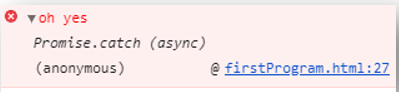##### JavaScript Chaining Promises flow

Each then() method returns a new promise. The value of the promise is:

1. The return value of the success handler, if the previous promise is fulfilled
2. The error data if the previous promise failed
##### Multiple handlers for Promise

When we call the then() method multiple times on a promise then it is not promise chaining. Please have a look at the following example for a better understanding.

```<html>
<title>JavaScript Multiple then() handlers for Promise example</title>
<body>
<script>
let p = new Promise((resolve, reject) => {
setTimeout(() => {
resolve(5);
}, 3 * 1000);
});

p.then((result) => {
console.log(result); // 5
return result * 2;
})

p.then((result) => {
console.log(result); // 5
return result * 3;
})

p.then((result) => {
console.log(result); // 5
return result * 4;
});

p.then((result) => {
console.log(result); // 5
return result * 5;
});
</script>
</body>
</html>
```

Output: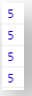In the above multiple handlers’ example, we see that we have multiple handlers for one promise. These handlers have not a relationship between them. Thus they execute independently and also they don’t pass the result from one then() to another then() as we saw in the promise chaining above.

##### The following picture illustrates a promise that has multiple handlers: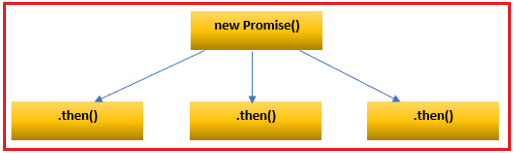##### Returning a Promise

When we return a value in the then() method, the then() method returns a new Promise that immediately resolves to the return value. Also, we can return a new promise in the then() method, like the below example:

```<html>
<title>JavaScript Returning a new Promise example</title>
<body>
<script>
let p = new Promise((resolve, reject) => {
setTimeout(() => {
resolve(5);
}, 3 * 1000);
});

p.then((result) => {
console.log(result);
return new Promise((resolve, reject) => {
setTimeout(() => {
resolve(result * 2);
}, 3 * 1000);
});
}).then((result) => {
console.log(result);
return new Promise((resolve, reject) => {
setTimeout(() => {
resolve(result * 3);
}, 3 * 1000);
});
}).then((result) => {
console.log(result);
return new Promise((resolve, reject) => {
setTimeout(() => {
resolve(result * 3);
}, 3 * 1000);
});
}).then(result => console.log(result));
</script>
</body>
</html>
```

Output: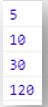The above example will return a result as 5,10,30 and 120 after every 3 seconds. The above code pattern for returning a promise allow use to execute some tasks in sequence. The above example of returning a promise code can be refractor as below:

```<html>
<title>JavaScript Returning a Promise refractor example</title>
<body>
<script>
function generateNumSeq(num) {
return new Promise((resolve, reject) => {
setTimeout(() => {
resolve(num);
}, 3 * 1000);
});
}

generateNumSeq(5)
.then(result => {
console.log(result);
return generateNumSeq(result * 2);
})
.then((result) => {
console.log(result);
return generateNumSeq(result * 3);
})
.then((result) => {
console.log(result);
return generateNumSeq(result * 4);
})
.then(result => console.log(result));
</script>
</body>
</html>
```

Output: